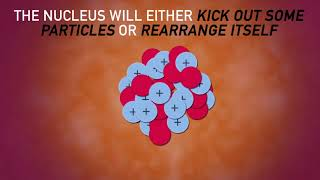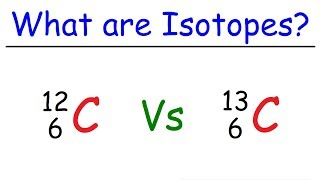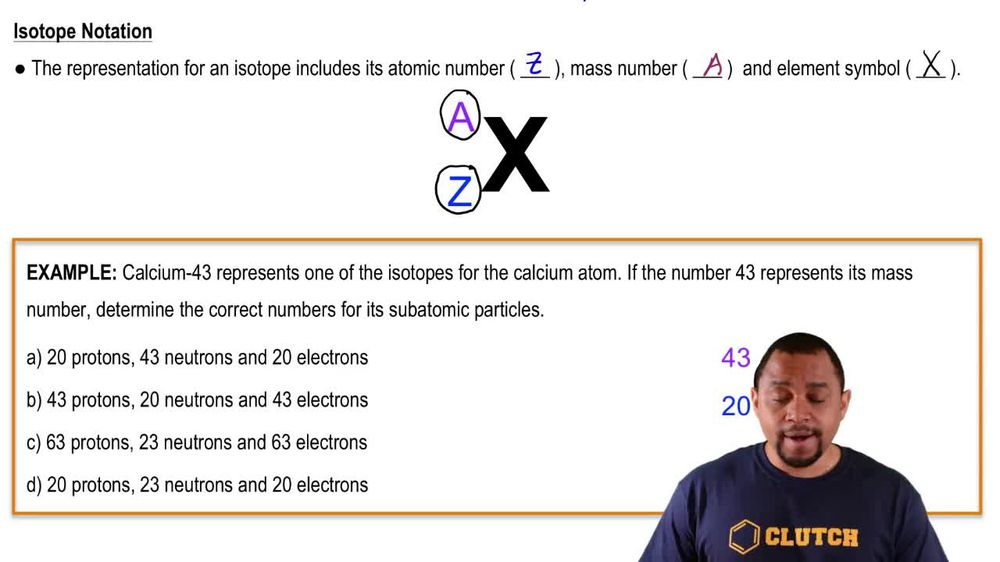Start typing, then use the up and down arrows to select an option from the list.
1. 2. Atoms & Elements2. Isotopes
Problem

# Fluorine occurs naturally as a single isotope. How many protons, neutrons, and electrons are present in deuterium fluoride (2HF)? (Deuterium is 2H.)

Relevant Solution2m
Play a video:
Hey everyone, we're told that sodium has only one naturally occurring isotope. While bromine has two naturally occurring isotopes, bromine 79 and roaming 81, determine the number of protons neutrons and electrons present in sodium bromide, 81. To answer this question, let's break it down per element starting with sodium To determine the number of protons. We can simply look at our atomic number since that tells us our number of protons and when we look at our periodic table, we find that this is 11 To determine the number of electrons. This is also going to be 11 since we have a neutral atom. Now for our mass number, we can also find this by looking at our periodic table and we find that it is 23 to determine the number of neutrons, We can simply take our mass number which is 23 and subtract the number of protons. So we subtract 11, which gets us to a value of 12 neutrons. Now looking at Bro mean 81, we can take the same steps. When we look at our periodic table, Our atomic number for browning is going to be 35. So the number of protons is 35. Again, since we have a neutral atom, the number of electrons is also going to be 35. Looking at our maths number in our periodic table, we find that it is 81 and to determine the number of neutrons. Again, we will take our mass number And subtract our number of protons. So we have 81 -35, which gets us to a value of 46. Now we can go ahead and calculate the total from these two elements, starting with the number of protons, We're going to take 11 and add this onto 35, which gets us to a total of 46. Looking at our number of electrons again, we're going to take 11 and add this to 35, which will also be 46. And lastly looking at the number of neutrons We can go ahead and take that value of 12 from our sodium and add this on to 46, which is from our browning 81. This gets us to a total value of 58 and this is going to be our final answers. Now, I hope that made sense. And let us know if you have any questions.